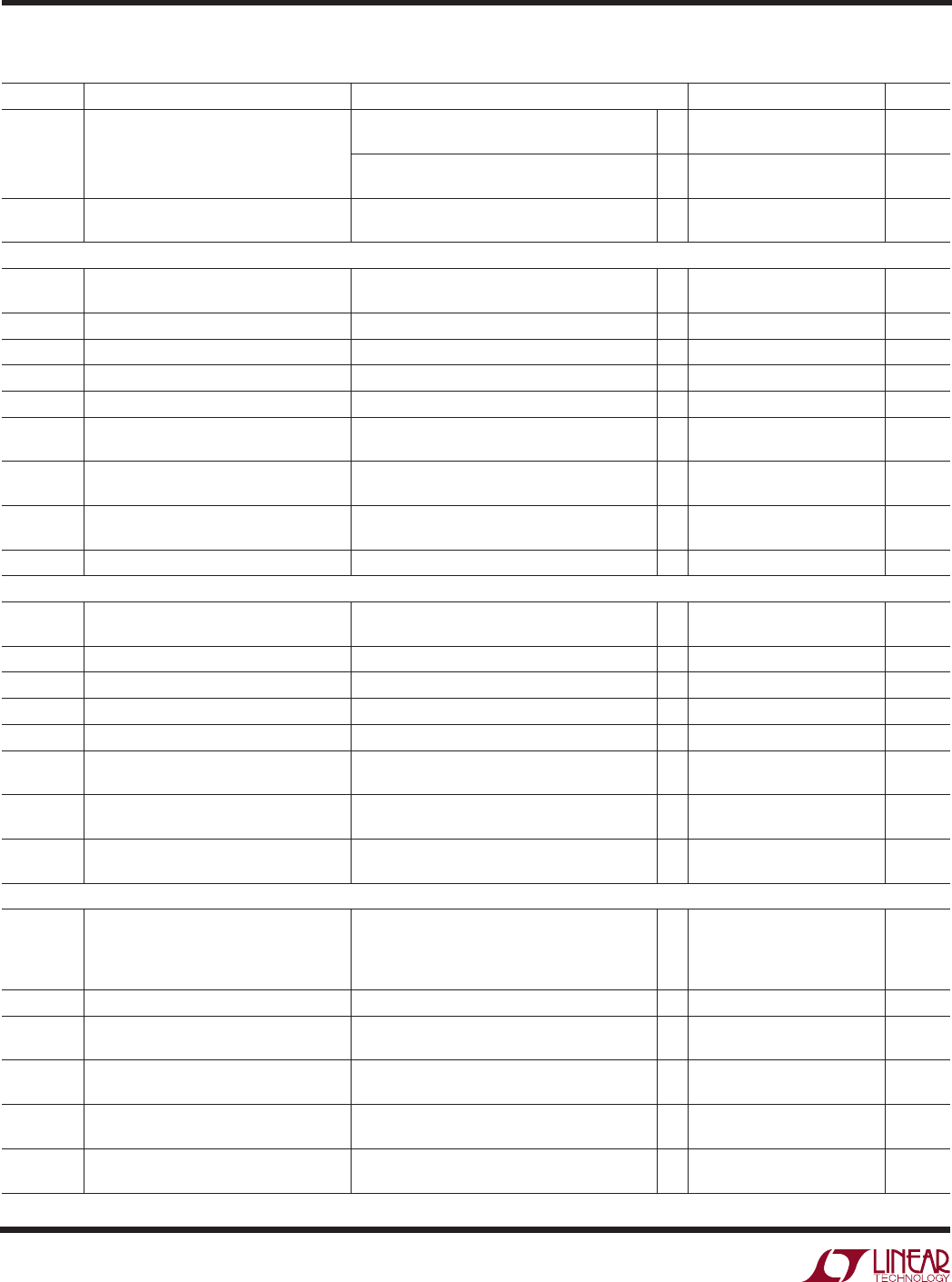Datasheet4
LTC1343
ELECTRICAL CHARACTERISTICS
The denotes specifications which apply over the full operating
temperature range, otherwise specifications are at T
A
= 25°C. V
CC
= 5V (Notes 2, 3)
SYMBOL PARAMETER CONDITIONS MIN TYP MAX UNITS
t
PHL
Input to Output (Figures 2, 7), CTRL = GND, 0°C T
A
70°C 35 80 115 ns
CTRL = V
CC
, 0°C T
A
70°C 400 ns
(Figures 2, 7), CTRL = GND, –40°C T
A
85°C 25 80 130 ns
CTRL = V
CC
, –40°C T
A
85°C 400 ns
t Input to Output Difference, t
PLH
– t
PHL
(Figures 2, 7), 0°C T
A
70°C 0517 ns
(Figures 2, 7), –40°C T
A
85°C 0525 ns
V.35 Driver
V
OD
Differential Output Voltage Open Circuit 6.0 V
CM
= 4.0V (Figure 3) ±0.44 ±0.55 ±0.66 V
I
OH
Transmitter Output High Current V
A, B
= 0V 12.6 11 9.4 mA
I
OL
Transmitter Output Low Current V
A, B
= 0V 9.4 11 12.6 mA
I
OZ
Transmitter Output Leakage Current 0.25V V
A, B
0.25V ±0.01 ±100 µA
t
r
, t
f
Rise or Fall Time (Figures 3, 6) 5 ns
t
PLH
Input to Output (Figures 3, 6), 0°C T
A
70°C 25 45 75 ns
(Figures 3, 6), –40°C T
A
85°C 25 45 90 ns
t
PHL
Input to Output (Figures 3, 6), 0°C T
A
70°C 25 45 75 ns
(Figures 3, 6), –40°C T
A
85°C 25 45 90 ns
t Input to Output Difference, t
PLH
– t
PHL
(Figures 3, 6), 0°C T
A
70°C 0517 ns
(Figures 3, 6), –40°C T
A
85°C 0525 ns
t
SKEW
Output to Output Skew (Figures 3, 6) 4 ns
V
TH
A
+ V
B
)/2 2V (Figure 3) 0.2 0.2 V
Threshold Voltage
V
TH
A
+ V
B
)/2 2V (Figure 3) 11 40 mV
I
IN
Receiver Input Current (A, B) 10V V
A, B
10V ±0.50 mA
R
IN
A, B
10V 20 32 k
t
r
, t
f
Rise or Fall Time (Figures 3, 7) 15 ns
t
PLH
Input to Output (Figures 3, 7), 0°C T
A
70°C 80 115 ns
(Figures 3, 7), –40°C T
A
85°C 80 130 ns
t
PHL
Input to Output (Figures 3, 7), 0°C T
A
70°C 100 115 ns
(Figures 3, 7), –40°C T
A
85°C 100 130 ns
t Input to Output Difference, t
PLH
– t
PHL
(Figures 3, 7), 0°C T
A
70°C 417 ns
(Figures 3, 7), –40°C T
A
85°C 425 ns
V.10 Driver
V
O
Output Voltage Open Circuit, R
L
= 3.9k ±4.0 ±6.0 V
R
L
= 450 (Figure 4) ±3.6 V
V
O
at 450 > 0.9 V
O
at R
L
= 3.9k
Driver 1 Only
I
SS
Short-Circuit Current V
O
= GND; EIA-530, X.21, EIA-530-A Modes ±150 mA
I
OZ
Output Leakage Current 0.25V V
O
0.25V, Power Off or ±0.1 ±100 µA
No-Cable Mode or Driver Disabled
t
r
, t
f
Rise or Fall Time (Figures 4, 8), R
L
= 450, C
L
= 100pF
R
423SET
= 100k 4 µs
t
PLH
Input to Output (Figures 4, 8), R
L
= 450, C
L
= 100pF
R
423SET
= 100k 8 µs
t
PHL
Input to Output (Figures 4, 8), R
L
= 450, C
L
= 100pF
R
423SET
= 100k 8 µs